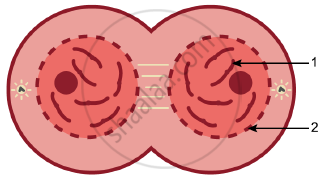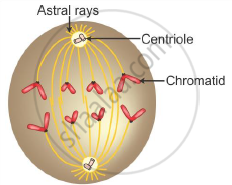Share

# Study the diagram given below which represents a stage during the mitotic cell division and answer the questions that follow - ICSE Class 10 - Biology

ConceptSignificance and Major Differences Between Mitotic and Meiotic Division

#### Question

Study the diagram given below which represents a stage during the mitotic cell division and answer the questions that follow(i) Identify the stage giving suitable reasons.
(ii) Name the parts numbered 1 and 2.
(iii) What is the technical term for the division of nucleus?
(iv) Mention the stage that comes before the stage shown in the diagram. Draw a neat labelled diagram of the stage mentioned.
(v) Which is the cell division that results in half the number of chromosomes in daughter cells?

#### Solution

(i) Telophase
(ii) 1 – Daughter chromosome, 2 – Nuclear membrane
(iii) Karyokinesis is the technical term for the division of a nucleus.
(iv) Anaphase(v) Meiosis

Is there an error in this question or solution?

#### APPEARS IN

Solution Study the diagram given below which represents a stage during the mitotic cell division and answer the questions that follow Concept: Significance and Major Differences Between Mitotic and Meiotic Division.
S